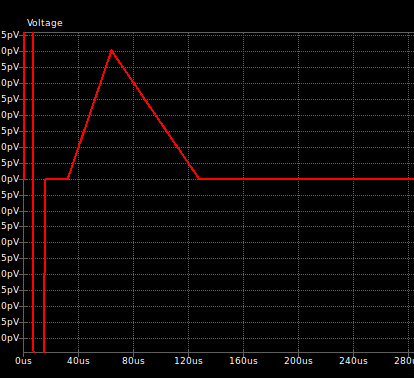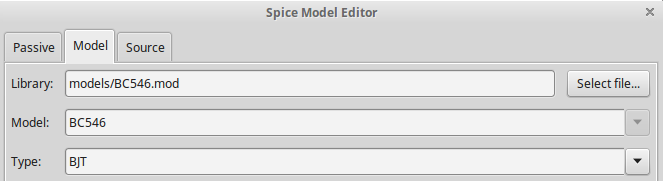# [SOLVED] Need help with Ngspice official BJT example for KiCad

I am working through the following guide:
http://ngspice.sourceforge.net/ngspice-eeschema.html#BipAmp

I’ve had no problem with steps 2 and 4, but I can’t get step 3 to work (BJT simulation). I’ve reproduced the exact same schematic as the screenshot provided in the guide under Eeschema, using only PSPICE devices, except the BC546 which comes from Transistor_BJT. All component values are identical to the screenshot too.

I’ve tried the three models with identical results (both links from the guide, and this one: https://www.diyaudio.com/forums/software-tools/278096-bc546-spice-model.html#post5587810). I’ve tried all permutations of “alternate node sequences” (i.e. pinouts, as described at step 4). I’ve simulated with both a ‘sin(0 1m 500)’ input and a fixed 0V input. Regardless of the input signal, the output is always a short swing in the pV range followed by a 0V flatline. I am using KiCad 5.1.5 (under Linux) and the embedded version of Ngspice (ngspice-31 i believe?)

Can someone help me figure out what I’m not doing right?

After you have run the simulation, do the following:
Tools->Simulator->Simulation->Show spice netlist…
Mark and copy the netlist and post it here.

In addition to the netlist, can you try taking a screenshot (of the schematic) and pasting it within your response? If it doesn’t let you because you’re new, try a file hosting website and shoot us a link. I suspect a schematic drawing error, and it’ll be easier to pick out visually.

Thanks so much for the support. This is my spice netlist:

``````.title KiCad schematic
Vcc Vcc 0 5
Vin in 0 sin(0 1m 500)
.save @r1[i]
.save @r2[i]
.save @c1[i]
.save @c2[i]
.save @vcc[i]
.save @r3[i]
.save @q1[ib]
.save @q1[ic]
.save @q1[ie]
.save @vin[i]
.save V(Vcc)
.save V(in)
.save V(out)
.tran 100u 10m
.end
``````

Screenshot:

I’m not sure what’s going on. Your netlist (besides the copy/paste screenshot error in the .include line) looks fine to me and simulates fine for me too. Schematic looks good too. I suspect something with how the model is used since I don’t have access to your `BC546.mod` that’s being referenced. I attached a ZIP of my version of the project in case it helps you figure out what the discrepancy is. If you have the ability to ZIP your own project and upload it, I can take a look at your project as a whole too.

bjt_example.zip (4.7 KB)

1 Like

Normally this circuit also has a resistor between the emitter and GND.
(A few hundred Ohms will do. It’s value should be lagger then: R2/Hfe ).

Without this, the base will be “stuck” at 600mV, and the transistor will go into saturation easily, which will prevent it from working as a lineair amplifier.

Yes, but this is irrelevant to the problem at hand.

here:

``````* https://www.diyaudio.com/forums/software-tools/278096-bc546-spice-model.html#post5587810
.MODEL BC546B NPN (
+ IS=2.39E-14
+ NF=1.008
+ ISE=3.545E-15
+ NE=1.541
+ BF=294.3
+ IKF=0.1357
+ VAF=63.2
+ NR=1.004
+ ISC=6.272E-14
+ NC=1.243
+ BR=7.946
+ IKR=0.1144
+ VAR=25.9
+ RB=1
+ IRB=1E-06
+ RBM=1
+ RE=0.4683
+ RC=0.85
+ XTB=0
+ EG=1.11
+ XTI=3
+ CJE=1.358E-11
+ VJE=0.65
+ MJE=0.3279
+ TF=4.391E-10
+ XTF=120
+ VTF=2.643
+ ITF=0.7495
+ PTF=0
+ CJC=3.728E-12
+ VJC=0.3997
+ MJC=0.2955
+ XCJC=0.6193
+ TR=1E-32
+ CJS=0
+ VJS=0.75
+ MJS=0.333
+ FC=0.9579 )
``````

I previously also tried

``````*From Philips SC04 "Small signal transistors 1991"
* Base spreading parameters (RB,IRB,RBM) estimated. TR derived using BCY58 data
.model BC546B npn ( IS=7.59E-15 VAF=73.4 BF=480 IKF=0.0962 NE=1.2665
+ ISE=3.278E-15 IKR=0.03 ISC=2.00E-13 NC=1.2 NR=1 BR=5 RC=0.25 CJC=6.33E-12
+ FC=0.5 MJC=0.33 VJC=0.65 CJE=1.25E-11 MJE=0.55 VJE=0.65 TF=4.26E-10
+ ITF=0.6 VTF=3 XTF=20 RB=100 IRB=0.0001 RBM=10 RE=0.5 TR=1.50E-07)
``````

It’s a freestanding Eeschema file. As a new user, I can’t attach it directly here but here you go: https://we.tl/t-UsiWTvEYQt

I tried simulating with a 500Ω resistor in that position but no difference.

FWIW, this is the output I get. The initial swing peaks just above 129pV, then -32pV, then what’s on screenshot (below). There is also a linear rise from 0V to roughly 2pV during the last 100ns simulated or thereaboutsThat’s all great, but what happens when you run the project I posted?

I downloaded and ran the bjt_example posted here, and it works for me without modification.
Just opened the project, and opened Eechema, then ran the simulator and added V(in) and V(out) signals to the plot.

If you see a plot result in pV or some other nonsensical stuff, it’s usually an error in the schematic or the model, or with pin mapping, version incompatibilies, etc.

When experimenting with something you do not have much experience with, it’s always nice to have a working example such as the bjt_example as posted here.

I do recall something about installing a newer version of ngspice to get it working in KiCad, but 2 weeks ago my regular PC crashed and I’m now typing this on an intermediate, and i’m not sure what exactly is installed here, or if it’s still needed to update ngspice manually (on linux) with the latest stable KiCad version.

I loaded your Eeschema file and simulated it, it worked fine. I opened the model editor for the BJT and found this SPICE code:

``````.model BC546B npn ( IS=7.59E-15 VAF=73.4 BF=480 IKF=0.0962 NE=1.2665
+ ISE=3.278E-15 IKR=0.03 ISC=2.00E-13 NC=1.2 NR=1 BR=5 RC=0.25 CJC=6.33E-12
+ FC=0.5 MJC=0.33 VJC=0.65 CJE=1.25E-11 MJE=0.55 VJE=0.65 TF=4.26E-10
+ ITF=0.6 VTF=3 XTF=20 RB=100 IRB=0.0001 RBM=10 RE=0.5 TR=1.50E-07)
``````

I copied it into a new .mod file and loaded it for the BJT in my schematic, it made no difference.

What happened when you ran mine?

Since you only sent a `.sch` file without any supporting files, naturally it’s going to do this:If I copy my own `.mod` file to the same directory and redefine where KiCad looks for it, then it works just like my own project.

Sorry I didn’t know that. Here is the zipped up folder, including the generated project files (both your schematic and mine), as well as some models I’ve tried: https://we.tl/t-HaJ7CGJsok

Strangely, I’ve tried BC546.2.mod (also in zip file) in your project and it works fine too. Your findings point to problems with my model; my findings point to problems with the schematic file?

Not sure…if I open your schematic, add the B to end of the `Spice_Model` field (because it’s missing), and then simulate, it works fine for me.

Does that give any useful clues?

1 Like

That’s what’s wrong with my schematic: adding the B hence referring to the correct spice model fixed it. The tutorial specifically points to a BC546B model and makes no mention of this extra step. As a total beginner it threw me off. Might be worth explaining in the tutorial ?

Anyway, problem solved, thanks so much for your help!

It’s not an extra step. You’re supposed to load up the library file, and then select the BC546B from the drop-down selector. I have no idea where your lack of B came from. That drop-down auto-populates with items from all the `.model` and .`subckt` statements found within the selected library file.

That drop down is greyed out for me till I add the ‘B’ manually. It’s available once I’ve done that.I’m unable to duplicate that. There shouldn’t be anything manually entered in there in the first place. What happens if you delete the transistor from the schematic and place a new fresh one down? Do you still get the same behavior?

I can’t reproduce it myself anymore either? Not sure how my schematic got into that state